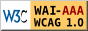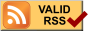# Scheme note

## 簡介(Introduction)

• Scheme 是1975年誕生於MIT 人工智慧實驗室的一門程式語言。它是一套函數式(functional)的設計語言，由LISP衍生而來，主要應用在人工智慧這個領域上，或是結構相當地複雜難以用傳統語言描述之，擅長處理符號。
• Scheme和 LISP 是很有淵源的都屬於函數語言 (functional programming languages)意思是說,盡量把所有的函數都想成/寫成數學上的函數。函數語言都以lambda calculus 為理論基礎。
• Scheme被稱為程式語言的皇后。
• Scheme 是弱型別語言(weakly typed language)，變數本身沒有型別，綁在變數上的值才有型別， 而不是像 C 一樣，型別跟著變數走。
• Scheme is a weakly typed language with dynamic type checking and lexical scope rules.
• Scheme 與Java 一樣都具有記憶體回收機制(garbage collection)。
• 函數和整數、字串一樣，也是一種資料型態，稱之為函數型別。

### Scheme語言的標準

#### R5RS(Revised(5) Report on the algorithmic language Scheme)

R5RS 為現有Scheme語言的標準，於1998年制訂 Scheme語法規則的第5次修正

### Scheme語言的實作版本

Scheme 是一種 Lisp 方言，目前的規範是 R5RS，和 C 語言一樣，同樣的規格書有不同的實作， 每個實作在標準之上可以再加一些特色或函式庫等。

## 語法(Syntax)

• 整個scheme可以說是 read-eval-print loop 的運作方式：即讀取，計算，印出 的過程。
• scheme沒有大小寫之分
• 由函數組合所構成，可以巢狀組合，沒有main這個主函數進入點，以小括號將運算式括起來，函數名稱或運算元在左括號的右邊，運算子彼此以空白為間隔，如3+4*5這個運算式以Sheme語法撰寫如： (+ 3 (* 4 5)) ，類似資料結構中的前序運算式。
• 基本的資料型態為原子 ( atom ) 及字串 ( list )。
• 原子 ( atom ) 包含符號 ( symbol ) 及數值 ( number ) 。
• 串列 ( list ) 則是以小括號括起來的一串資料。

### 註解

Scheme的標準中僅有單行註解：「; 」(分號) ，但實作的眾多Scheme版本卻幾乎都有多行註解，例如Guile的 「#! 」，「 !# 」(一定要分2行)

#### 以scheme作script語言

#! /usr/local/bin/guile -s
!#

### 定義變數

#### (define idexp)

(define pi 3.14159)

### 變數設值

#### (set! idexp)

(set! pi "hello")

## 資料型態(Types)

Scheme的資料型態如同python,perl,VBscript一樣，可隨時變更變數的資料型態

### 單一型態(Simple Types)

• boolean：#t , #f
> (not #f)
true
• number ： 依照數學原理來進行分類
• 包含了整數(integer)，有理數(rational)，實數(real)，複數(complex)等
• 若按照進制分類則有二進制(#b123)，八進制(#o123)，十進制(#d123)，十六進制(#x123)
• 除了一般的+,-,*之外，除法分整數除法 quotient 與實數除法 /
• 範例
1. (+ 4 5.2 7.5 2.0)
2. (/ 81 3 3 3)
3. (* '5 '7)
4. (quotient 9 4)
• char：字元以「#\」為前導，如#\A, #\8, #\%
• symbol：

### 複合型態(Composite Types)

#### string：由多個字元所組成

>(define name "peter")　;定義字串變數name
>name
"peter"
>(string-length name) 　;字串變數name的長度
5
>(string-ref name 3) 　;字串變數name第3個位置的字元
#\e

#### list (串列)：在Scheme中的基本結構資料型態，其內的資料型態不必相同

• ()：空串列，稱為NIL
• list : 將參數列轉為list
• (list 'a 'b 'c) => (a b c)
• (list '(a b c) '(d e f)) => ((a b c)(d e f))
• quote / ' : 將list視為資料而不評估它
• (quote (a b c)) => (a b c)
• (quote (+ 3 4)) => (+ 3 4)
• (quote (quote(+ 3 4))) => '(+ 3 4)
• car : returns the first element of a list(從list之中抓出第一個成員)
• (car '(1 2 3)) => 1
• (car '((a b) (c d)) => (a b)
• (car '(first second third)) => first
• cdr : returns the list without first element(去除第一個成員後的list)
• (cdr '(1 2 3)) => (2 3)
• (cdr '((a b) (c d)) => ((c d))
• (cdr '(first second third)) => (second third)
• cons :
• takes two elements and returns a list(將前面的成員推入後面的list)
• (cons '1 '(2 3 4)) => (1 2 3 4)
• (cons '(1 2 3) '(4 5 6)) => ((1 2 3) 4 5 6)
• takes two elements and returns a pair(若後面非list,則2個成員將組成一個pair)
• (cons 'a 'b) => (a . b)
• 結合2個list ：(append '(1 2 3) '(4 5 6))  => (1 2 3 4 5 6)
• 反轉一個list ：(reverse '(1 2 3))  => (3 2 1)

### 檢查型態為何(Type Predicates)

• 是否為字串：(string? "5")
• 是否為數字：(number? (car (list 5 6)))
• 整數是否為偶數：(even? (car (list 5 6)))
• 整數是否為奇數：(odd? (car (list 5 6)))
• 實數是否為正數：(even? (car (list 5.7 6)))
• 實數是否為負數：(odd? (car (list -5.7 6)))
• 是否為0：(zero? (car (list 5 6)))
• 是否為邏輯值：(boolean? 5)
• 是否為null：(null? (cdr '(a)))

## 敘述句與流程控制

### 邏輯運算式(Boolean Expressions)

1. (< 5 6) (<= 5 6) (= 5 6) (> 5 6) (>= 5 5)
2. (equal? "abc" "abc") //是否資料的內容完全相同
3. (eq? "abc" "abc") //是否指向同一個物件

### 條件運算式(Conditional Expressions)

1. if : 單一判斷
(if (> 6 5) ; 判斷式
(* 3 5) ; 為真
(* 8 9)) ; 為假
2. cond : 多重判斷
(cond
((< n 0) 'lower)
((> n 0) 'upper)
(else 'equal)
)

## 函數(Functions)與程序(Procedure)

### 定義一個函數或程序

#### (define idexp)

>(define (f2c t) (* 5/9 (- t 32)))

>(define singleton (cons 'sample null))
>singleton
(sample)

### 變數設值 (set!)

#### (set! idexp)

(set! pi "hello")

### 定義一個不具名的函數或程序

#### (lambda (id.....)exp)

• lambda 取代了define 函數名稱 的位置
• ((lambda (x) (+ x x)) (* 3 4))　=>　24
• ((lambda (x y) (+ x y)) 3 4)　=>　7

### 定義一個可傳入參數的的函數或程序(結合define與lambda)

#### 定義一個square的函數

(define square
(lambda
(x)

(* x x)))
(define (square x)

(* x x))
(square 5) 25

#### 定義一個reciprocal的函數

(define reciprocal
(lambda (n)
(if (= n 0)
"oops!" (/ 1 n)
)
)
)
(reciprocal (reciprocal 10)) 10

### (eval運算式)

eval可以接受list當參數，然後評估其值，list內的變數，只能參照外層設定

>(set! x 3 )
>(define (f x) (eval '(print x)))
>(f 4)
3

### (mapcar 函數 list1 list2 ... listn )

>(set! x 3 )
>(define (f x) (eval '(print x)))
>(f 4)
3

### (length list)

>(define x (list 3 4 5))
>(length x)
3

## 變數(Variable)

### (let (變數=變數的值的list)(運算式))

• 程式中需要變數來暫時存放運算的結果，並將這些變數放在list內
• 指定變數的list運算式中可包含另一個let敘述成為巢狀

(let
((x 3) (y 2))
(+ x y)
)

### ((lambda (變數)(運算式))變數的值)

#### 例如：x=3; y=2; x+y=?

(
(lambda (x y) (+ x y))
3 4
)

## 錯誤訊息

• variable n is not bound　; 變數n未定義
• unbound variable n 　　　; 變數n未定義

## 相關名詞

 imperative language 命令導向式語言 functional language 函數導向式語言 algebraic simplifications 代數的簡化 lambda calculus 微積分 descendent 後代子孫，衍生物 genesis 起源 dialect 同樣來源，方言的 parenthesis(paren.)/parenteses 小括號/複數型 bracket 括號或中括號 commas 逗號 quote 引號 semicolon 分號

## 網路資源 (Resource)|||||